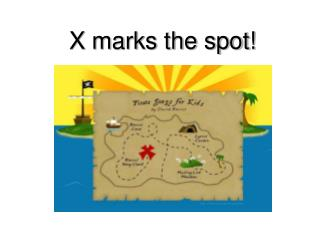# X marks the spot! - PowerPoint PPT PresentationDownload PresentationX marks the spot!

X marks the spot!Download Presentation## X marks the spot!

- - - - - - - - - - - - - - - - - - - - - - - - - - - E N D - - - - - - - - - - - - - - - - - - - - - - - - - - -
##### Presentation Transcript

1. X marks the spot!

2. The Coordinate Plane Lesson 1

3. Geometric quadrant with plumb bob. A quadrant is an instrument that is used to measure angles up to 90°.

4. The coordinate plane is formed by two number lines in a plane that intersect at right angles. The point of intersection is the zero on each number line.

5. 1 0 0 -1 1 -1

6. The two number lines are called the axes. The horizontal axis is called the x-axis. The vertical axis is called the y-axis.

7. The two axes divide the coordinate plane into four quadrants. The point where the axes intersect is called the origin.

8. Where am I? D Name the quadrant and the ordered pair for each point: A.__________ B.__________ C.__________ D.__________ E.__________ A C E B

9. Questions • Can someone tell me what are some of the common uses of the coordinate plane? http://www.miniclip.com/games/battleships/en/